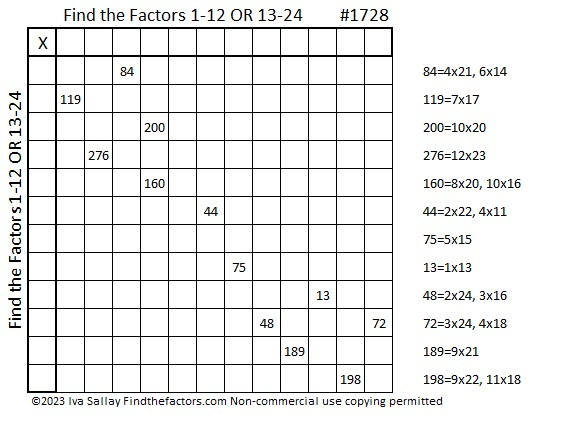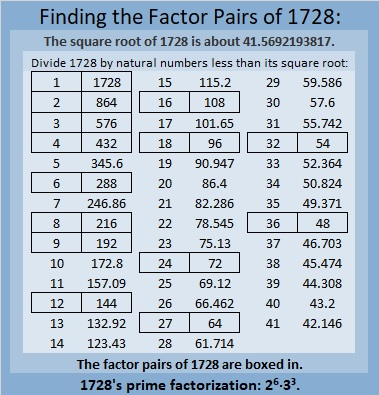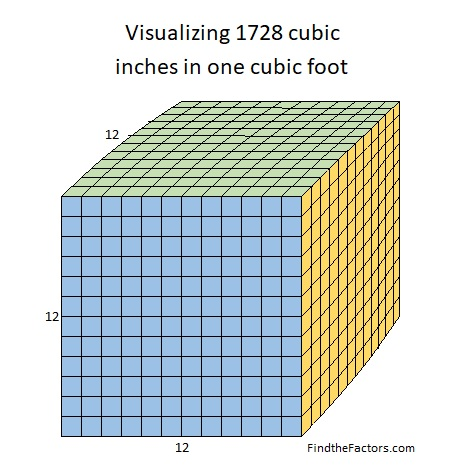# 1728 Is a Perfect Cube

Contents

### Today’s Puzzle:

I think this puzzle is a little bit harder than the previous two puzzles, so you might want to solve those two first. You will need to write all the numbers from 1 to 24 where the factors go, but keep the numbers from 1 to 12 together and the numbers from 13 to 24 together. There is only one solution. The possible factors are written to the right of the puzzle. Good luck!You can solve the puzzle by eliminating factors that must be used for other numbers. For example, 198 cannot be 9×22 because 189 must be 9×21, and the puzzle can only have one nine. Don’t write the factors on the multiplication table until after you know if the top row has the numbers 1 – 12 or if it has the numbers 13 – 24. The first column will then have the other set of numbers.

### Factors of 1728:

Let’s find out the factoring information of the puzzle number:

• 1728 is a composite number and a perfect cube.
• Prime factorization: 1728 = 2 × 2 × 2 × 2 × 2 × 2 × 3 × 3 × 3, which can be written 1728 = 2⁶ × 3³.
• 1728 has at least one exponent greater than 1 in its prime factorization so √1728 can be simplified. Taking the factor pair from the factor pair table below with the largest square number factor, we get √1728 = (√576)(√3) = 24√3.
• The exponents in the prime factorization are 6 and 3. Adding one to each exponent and multiplying we get (6 + 1)(3 + 1) = 7 × 4 = 28. Therefore 1728 has exactly 28 factors.
• The factors of 1728 are outlined with their factor pairs in the graphic below.### More About the Number 1728:

1728 is the 3rd smallest number with exactly 28 factors.

There are 1728 cubic inches in one cubic foot.12³₁₀ = 10³₁₂ (The cube above is 12 cubed in base 10, and it’s 10 cubed in base 12.)

1728 can be written as the sum of powers in some interesting ways:

2¹⁰ + 2⁹ + 2⁷ + 2⁶ = 1024 + 512 + 128 + 64 = 1728.
1(4⁵) + 2(4⁴) + 3(4³) = 1728.
1(6⁴) + 2(6³) = 1728.
3(8³) + 3(8²) = 3(8³ + 8²) = 1728.

11³ = 1331, and 1(11³) + 3(11²) + 3(11¹) + 1(11⁰) = 1728.

Here’s another connection with the number 11.
3(11²) = 363, and
1728 is palindrome 363 in base 23 because
3(23²) + 6(23¹) + 3(23⁰) = 1728.

1728 is 300 in base 24 because 3(24²) = 1728.

I’ve enjoyed researching the number 1728, and I hope you’ve learned some new and interesting fact about it today.

This site uses Akismet to reduce spam. Learn how your comment data is processed.